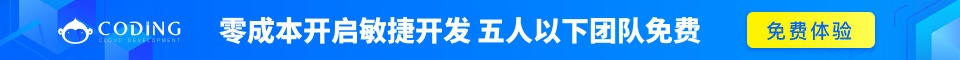# C 数组

C 语言支持数组数据结构，它可以存储一个固定大小的相同类型元素的顺序集合。数组是用来存储一系列数据，但它往往被认为是一系列相同类型的变量。## 声明数组

```type arrayName [ arraySize ];
```

```double balance;
```

## 初始化数组

```double balance = {1000.0, 2.0, 3.4, 7.0, 50.0};
```

```double balance[] = {1000.0, 2.0, 3.4, 7.0, 50.0};
```

```balance = 50.0;
```## 访问数组元素

```double salary = balance;
```

```#include <stdio.h>

int main ()
{
int n[ 10 ]; /* n 是一个包含 10 个整数的数组 */
int i,j;

/* 初始化数组元素 */
for ( i = 0; i < 10; i++ )
{
n[ i ] = i + 100; /* 设置元素 i 为 i + 100 */
}

/* 输出数组中每个元素的值 */
for (j = 0; j < 10; j++ )
{
printf("Element[%d] = %d\n", j, n[j] );
}

return 0;
}
```

```Element = 100
Element = 101
Element = 102
Element = 103
Element = 104
Element = 105
Element = 106
Element = 107
Element = 108
Element = 109
```